// This prints the left floatting menu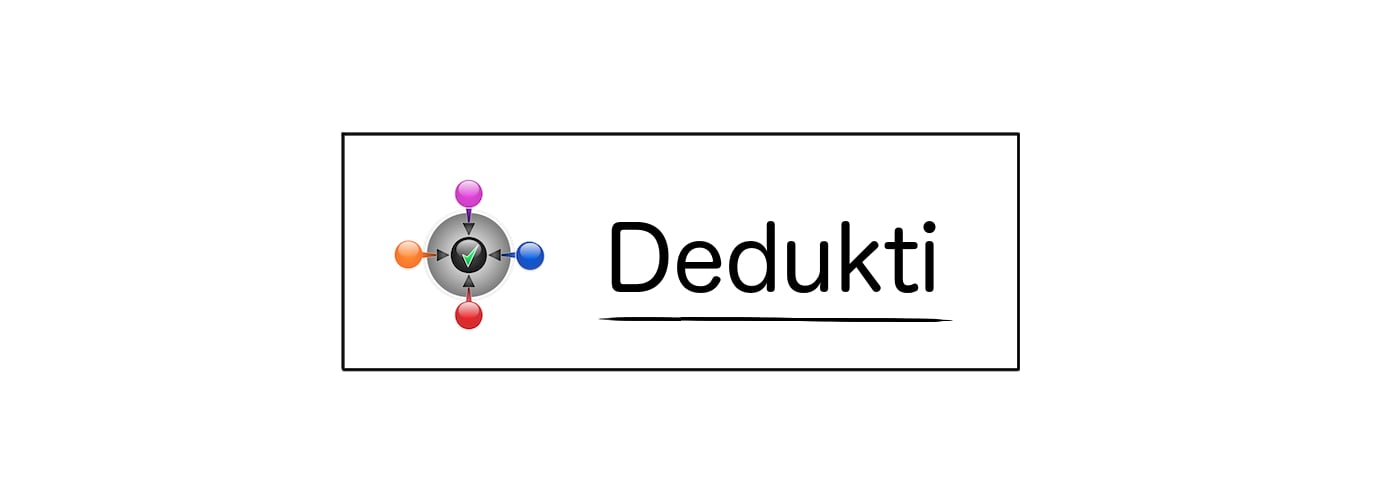### Theoremrelations.RC_reflexive

Statement

∀ R, reflexive (RC R)

Main Dependencies
Theory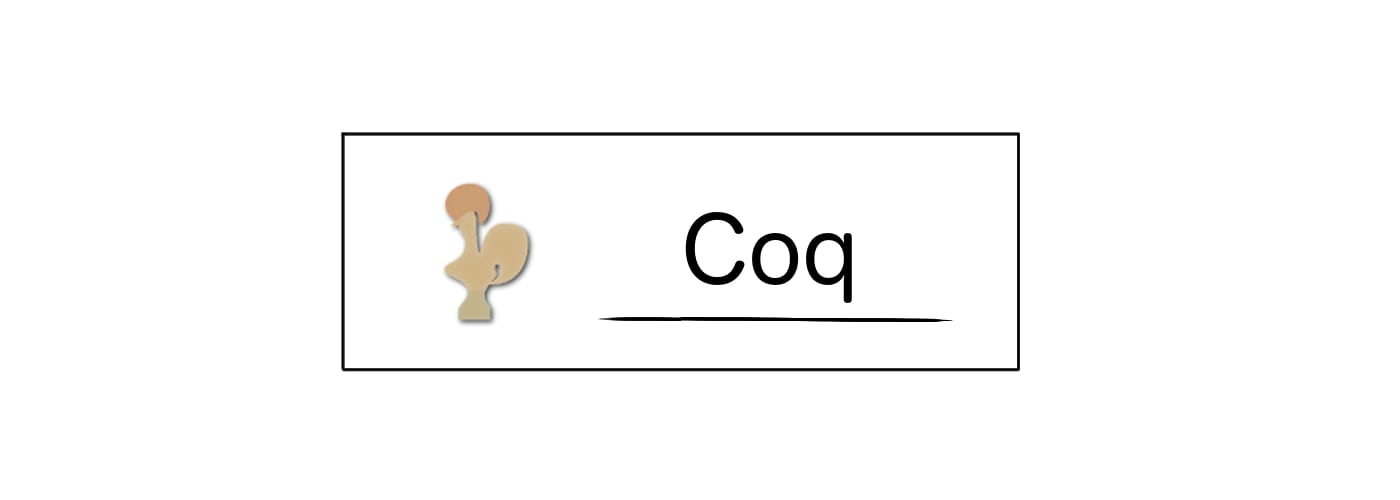Statement

Theorem RC_reflexive : forall A, forall (R:(A -> A -> Prop)), reflexive (A) (RC (A) R).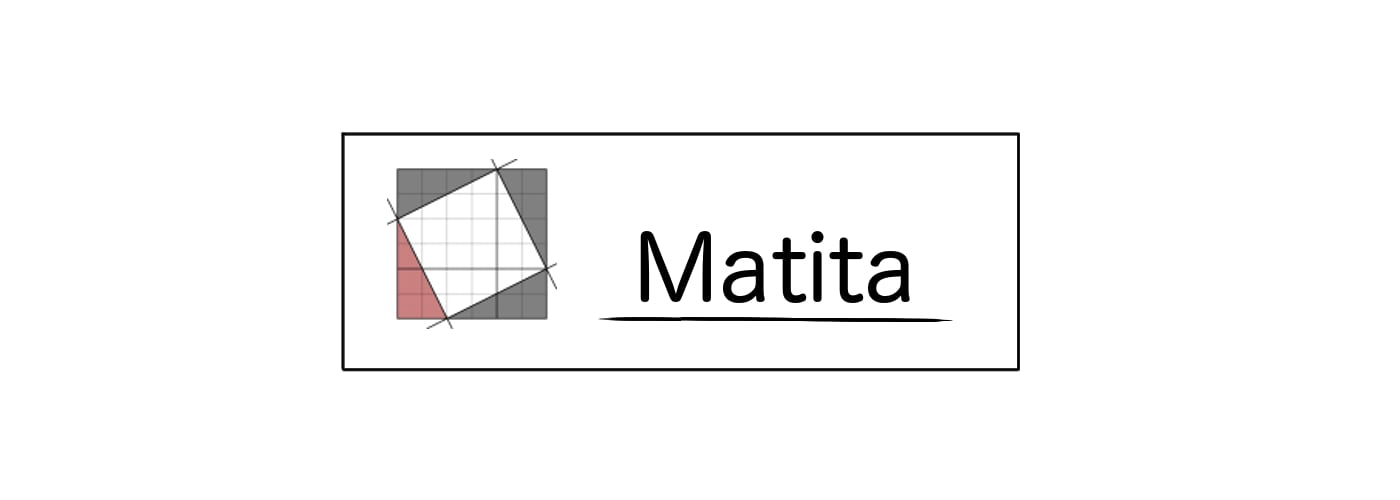Statement

theorem RC_reflexive : \forall A. \forall (R:A -> A -> Prop). (reflexive) (A) ((RC) (A) R).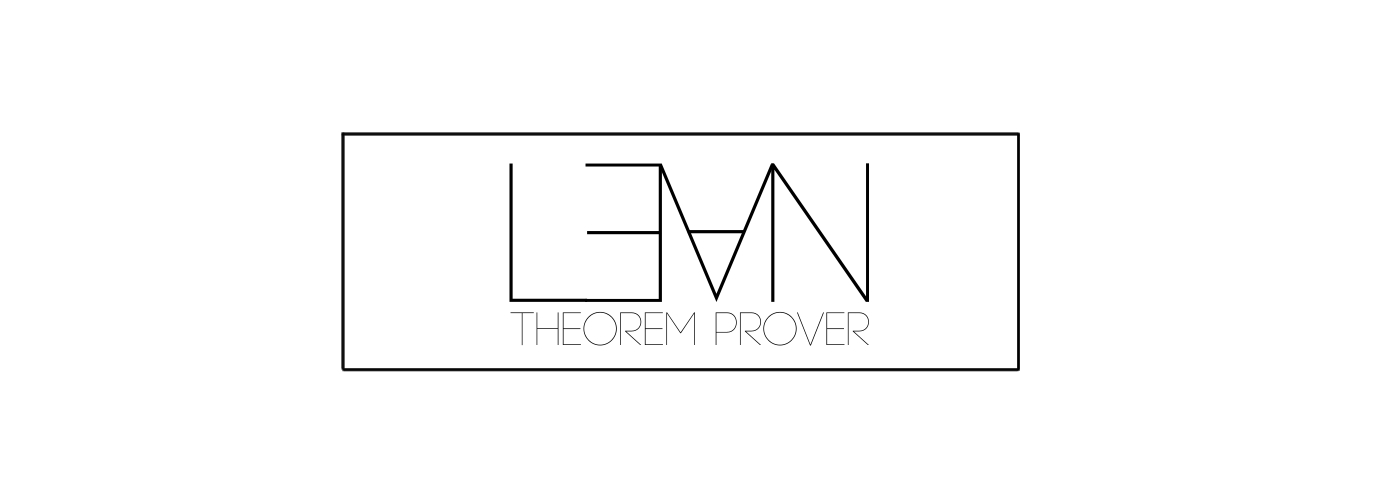Statement

theorem RC_reflexive : forall (A : Type) , forall (R:(A) -> (A) -> Prop) , ((relations.reflexive) (A)) (((relations.RC) (A)) (R)).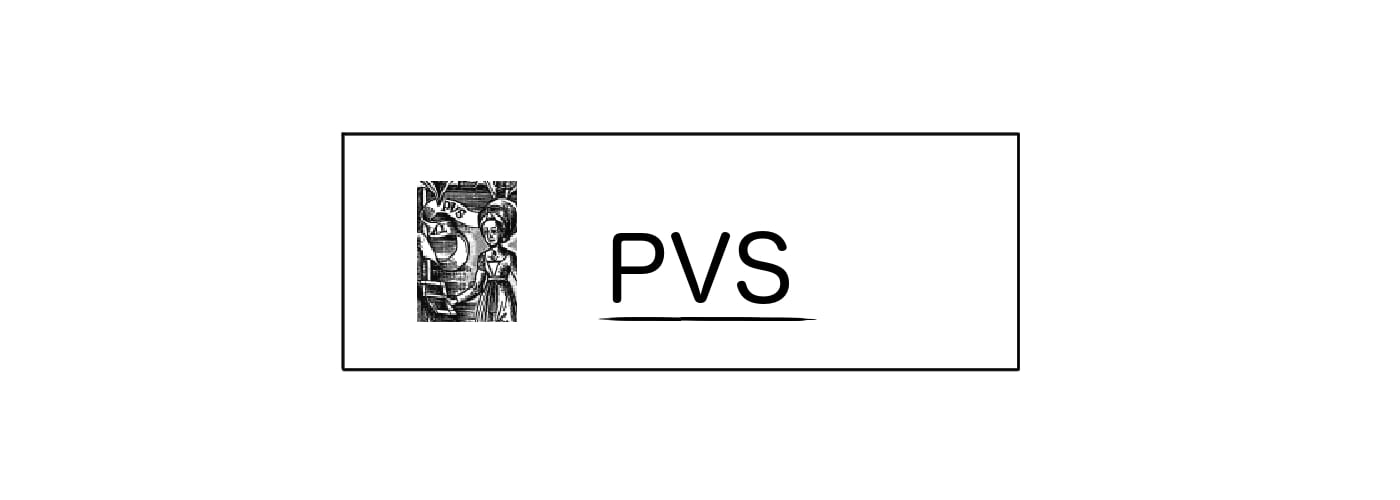Statement

RC_reflexive [A:TYPE+] : LEMMA (FORALL(R:[A -> [A -> bool]]):relations_sttfa.reflexive[A](relations_sttfa.RC[A](R)))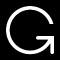Printing for OpenTheory is not working at the moment.